• 请参考：多元函数中可微可导的直观区别是什么、全微分 对于一元函数，可微和可导是一回事 对于多元函数来讲，可微指的是全微分，可导指的是偏导数 偏微分就好比过这一点的一个截面的切线，偏导数就是该切线的...
请参考：多元函数中可微与可导的直观区别是什么、全微分

对于一元函数，可微和可导是一回事

对于多元函数来讲，可微指的是全微分，可导指的是偏导数

偏微分就好比过这一点的一个截面的切线，偏导数就是该切线的斜率

全微分要求过这一点的所有的截面切线（360°无死角），共同所在的平面。

所以偏导数存在不一定存在全微分，但是反过来，如果多元函数可微，就一定可导。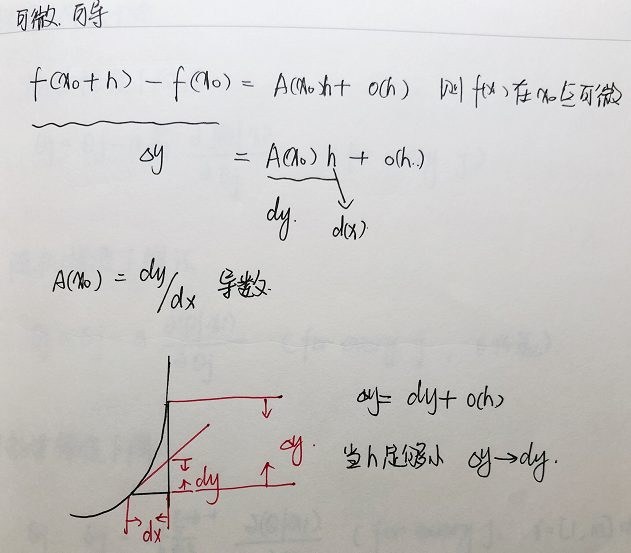展开全文• 偏微分是描述的局部细微变化，全微分整体上细微的变化，这里微分对应：细微变化，偏和全代表：局部，整体。应用在生活或者工业生产就是偏微分：单个条件对变化产生的影响。 全微分方程辨别；分别求偏导相等就是...


目录

全微分推导：

全微分感性理解：

全微分几何意义举例：

偏导与全微分的意义

通过物理性质理解。偏导与全微分的意义

全微分推导：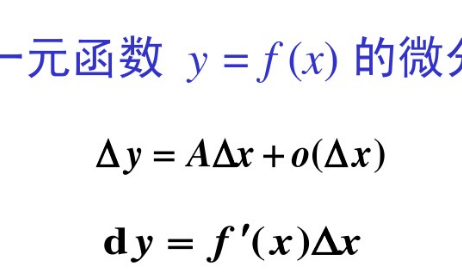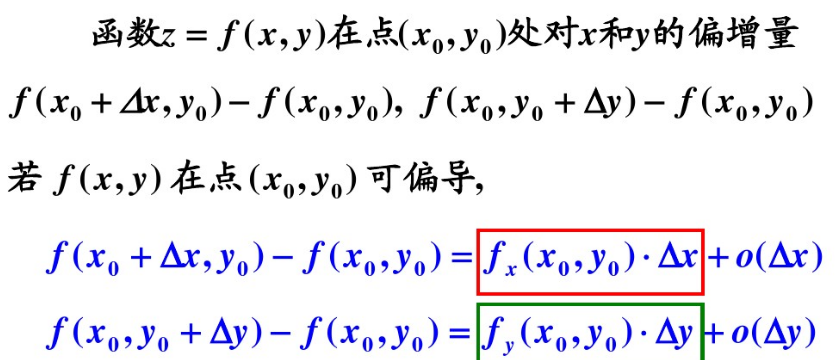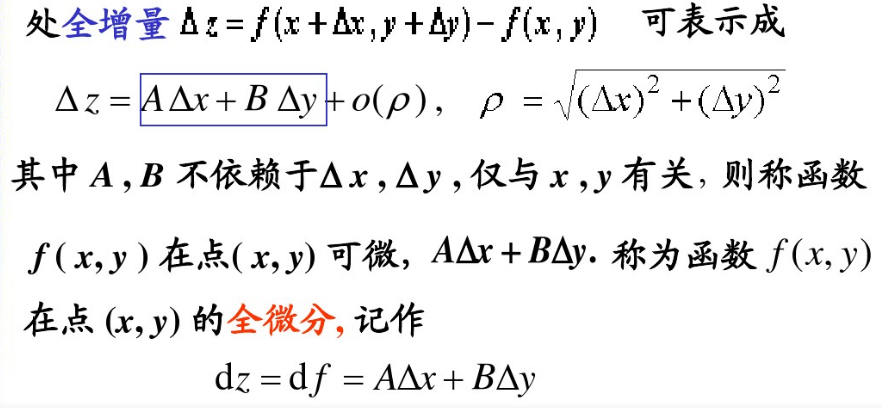全微分感性理解：

微分运行极限思想便能化曲为直；

一元函数微分就是切线；

二元函数全微分就是切面，也是无限分割，化曲为直；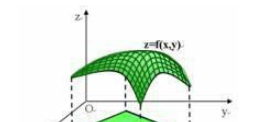对于z=f(x,y)偏导就是保持一个变量不变，另一个变量变化无限小，这时z的变化，这是就是一元函数导数；

全微分就是x，y都变化无限小，这是对于z=f(x,y)这个曲面，我们曲一小块正方形边长是：△x、△y;

这时的全微分就是关于这个小曲面的的切面；

全微分几何意义举例：

（zx：表示z关于x的偏导，zy：表示z关于y的偏导）

设z=xy，则两个偏导数分bai别为zx=y，zy=x。

所以，dz=zx·dx+zy·dy=ydx+xdy。

如果函du数z=f(x, y) 在(x, y)处的全增量Δz=f(x+Δx,y+Δy)-f(x,y)可以zhi表示为Δz=AΔx+BΔy+o(ρ)，

其中daoA、B不依赖于Δx, Δy，仅与x,y有关，ρ趋近于0(ρ=√[(Δx)2+(Δy)2])，此时称函数z=f(x, y)在点（x，y）处可微分，AΔx+BΔy称为函数z=f(x, y)在点(x, y)处的全微分，记为dz即dz=AΔx +BΔy。

该表达式称为函数z=f(x, y) 在(x, y)处(关于Δx, Δy)的全微分。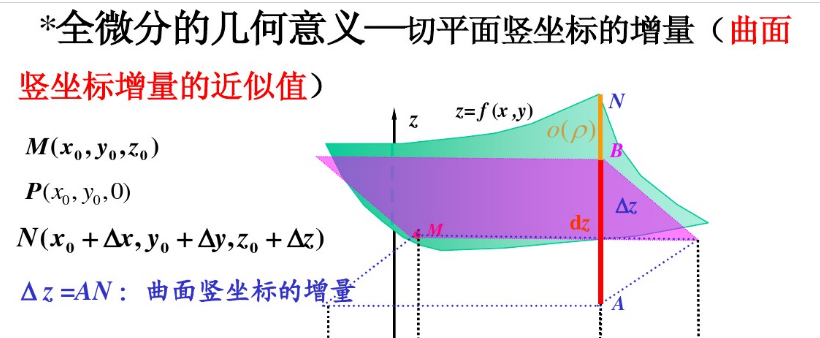dz==ydx+xdy :这里就是用切面的立标增量近似代替曲面立标增量；

偏导与全微分的意义

偏微分是描述的局部细微变化，全微

分整体上细微的变化，这里微分对应：细微变化，偏和全代表：局部，整体。应用在生活或者工业生产就是偏微分：单个条件对变化产生的影响。

全微分方程辨别；分别求偏导相等就是全微分方程。

（3x^2+6xy^2)dx+(4y^3+6x^2y)dy=0,P=3x^2+6xy^2,Q=4y^3+6x^2y,δP/δy=12xy=δQ/δx,所以这是全微分方程,

还有：

xydx+（x2+1）dy=0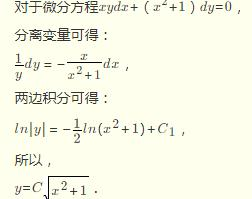通过物理性质理解。偏导与全微分的意义

1、偏导的物理意义：
单一参数的变化，引起的物理量的变化率。
例如：
A、∂P/∂T：温压变化率 = 压强随着温度的变化率；
B、∂V/∂T：体压变化率 = 体积随着温度的变化率。
.
2、全微分的物理意义：
所有参数同时变化，所引起函数的整体变化。
例如：
对于理想气体，P = nRT/V = f(T,V)
dP = (∂f/∂T)dT + (∂f/∂V)dV
也就是，
压强P的微小变化，是由温度引起的变化量(∂f/∂T)dT，
跟由体积引起的变化量(∂f/∂V)dV，这两者之和所确定。

1.偏导数
代数意义
偏导数是对一个变量求导,另一个变量当做数
对x求偏导的话y就看作一个数,描述的是x方向上的变化率
对y求偏导的话x就看作一个数,描述的是y方向上的变化率
几何意义对x求偏导是曲面z=f(x,y)在x方向上的切线
对y求偏导是曲面z=f(x,y)在x方向上的切线
这里在补充点.就是因为偏导数只能描述x方向或y方向上的变化情况,但是我们要了解各个方向上的情况,所以后面有方向导数的概念.
2.微分
偏增量：x增加时f(x,y)增量或y增加时f(x,y)
偏微分：在detax趋进于0时偏增量的线性主要部分
detaz=fx（x,y）detax+o(detax)
右边等式第一项就是线性主要部分,就叫做在（x,y）点对x的偏微分
这个等式也给出了求偏微分的方法,就是用求x的偏导数求偏微分
全增量：x,y都增加时f(x,y)的增量
全微分：根号(detax方+detay方）趋于0时,全增量的线性主要部分
同样也有求全微分公式,也建立了全微分和偏导数的关系
dz=Adx+Bdy 其中A就是对x求偏导,B就是对y求偏导
希望楼主注意的是导数和微分是两个概念,他们之间的关系就是上面所说的公式.概念上先有导数,再有微分,然后有了导数和微分的关系公式,公式同时也指明了求微分的方法.
3.全导数
全导数是在复合函数中的概念,和上面的概念不是一个系统,要分开.
u=a(t),v=b(t)
z=f[a(t),b(t)]
dz/dt 就是全导数,这是复合函数求导中的一种情况,只有这时才有全导数的概念.
dz/dt=(偏z/偏u)(du/dt)+(偏z/偏v)(dv/dt)
建议楼主在复合函数求导这里好好看看书,这里分为3种情况.1.中间变量一元就是上面的情况,才有全导数的概念.2.中间变量有多元,只能求偏导 3.中间变两有一元也有多元,还是求偏导.
对于你的题能求对x的偏导数,对y的偏导数,z的全微分,不能求全导数
如果z=f(x^2,2^x) 只有这种情况下dz/dx才是全导数!


展开全文• 学习到机器学习线性回归和逻辑回归时遇到了梯度下降算法，然后顺着扯出了一堆高数的相关概念理论：导数、导数、全微分、方向导数、梯度，重新回顾它们之间的一些关系，从网上和教材中摘录相关知识点。 通过函数...
学习到机器学习线性回归和逻辑回归时遇到了梯度下降算法，然后顺着扯出了一堆高数的相关概念理论：导数、偏导数、全微分、方向导数、梯度，重新回顾它们之间的一些关系，从网上和教材中摘录相关知识点。

通过函数的极限定义出导数(以一元函数为例)
函数f(x)在点x0可微的充分必要条件是函数f(x)在点x0处可导
扩展到多元函数时，衍生出偏导数

导数
定义：设函数$y=f(x)$在点$x_0$的某个领域内有定义，如果$\frac{Δy}{Δx}$在当$Δx$->0时极限存在，则称函数$y=f(x)$在$x_0$处可导，这个极限是函数$y=f(x)$在$x_0$处的导数
$f'(x_0)=\lim \limits_{Δx \to 0} \frac{Δy}{Δx}=\lim \limits_{Δx \to 0} \frac{f(x_0+Δx)-f(x_0)}{Δx}$
根据导数的定义，从某种意义上说导数的本质是一种极限

导数与导函数的关系是局部与整体的关系，导数通常是指一点，导函数则是指一个区间上的

在直线运动场景中，若x表示时刻，y表示距离，函数f表示时间与距离的关系$y=f(x)$,那么导数的含义就是在$x_0$时刻的瞬时速度
在直角坐标系中，$y=f(x)$表示一个曲线，导数的含义表示的是曲线在点$x_0$处的切线的斜率

微分
定义：设函数$y=f(x)$在某个领域内有定义，$x_0$及$x_0+Δx$在这区间内，如果增量
$Δy=f(x_0+x)-f(x_0)$
可表示为
$Δy=AΔx+o(Δx)$
其中A是不依赖$Δx$的常数，$o(Δx)$是指$Δx$趋于0时的高阶无穷小，那么称函数$y=f(x)$在点$x_0$是可微的，而$AΔx$叫做函数在点$x_0$相应于自变量增量$Δx$的微分，记作$\mathrm{d} y$，记作
$\mathrm{d}y=AΔx$

高阶无穷小的定义：如果$\lim \limits \frac{\beta}{\alpha}=0$，就说$\beta$是比$\alpha$高阶的无穷小，记作$\beta=o(\alpha)$

微分与导数的关系
上式$Δy=AΔx+o(Δx)$两边同时除以$Δx$得到
$\frac{Δy}{Δx}=A+\frac{o(Δx)}{Δx}$
当$Δx \to 0$时,上式左边就是导数的定义，而右边的$\frac{o(Δx)}{Δx}$因为是高阶无穷小，所以会趋向于0，得到以下等式
$A=\lim \limits_{Δx \to 0}\frac{Δy}{Δx}=f'(x_0)$
因此，如果函数$f(x)$在点$x_0$可微，则$f(x)$在点$x_0$也一定可导，且$A=f'(x_0)$，反之，如果$f(x)$在点$x_0$可导，存在下式
$\lim \limits_{Δx \to 0}\frac{Δy}{Δx}=f'(x_0)$
根据极限与无穷小的关系转化上式，当$Δx \to 0$时
$\frac{Δy}{Δx}=f'(x_0)+\alpha$
其中$\lim \limits_{Δx \to 0}a=0$，即$\lim \limits_{Δx \to 0}\frac{aΔx}{Δx}=0$,$aΔx=o(Δx)$，上式转化为下式(又回到了微分的定义)
$Δy=f'(x_0)Δx+o(Δx)$
因此，函数$f(x)$在点$x_0$可微的充分必要条件是函数$f(x)$在点$x_0$可导
$\mathrm{d}y=f'(x_0)Δx$
偏导数
一元函数的变化率是导数，多元函数的自变量有多个，当某个自变量x变化而其它自变量固定时，这时候对变化的自变量x进行求导，就称为多元函数对于x的偏导数。
定义：设函数$z=f(x,y)$在点$(x_0,y_0)$的某一领域内有定义，当$y$固定于$y_0$，而$x$在$x_0$处有增量$Δx$，相应的函数有增量
$f(x_0+Δx,y_0)-f(x_0,y_0)$
如果
$\lim \limits_{Δx \to 0}\frac{f(x_0+Δx,y_0)-f(x_0,y_0)}{Δx}$
存在，则称该极限为$z=f(x,y)$在点$(x_0,y_0)$处对$x$的偏导数
偏导数的几何意义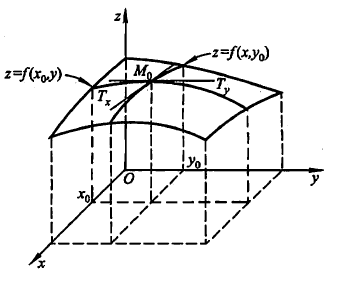偏导数$f_{x} (x_{0},y_{0} )$就是曲面被平面$y=y_{0}$所截得的曲线在点$M_{0}$处的切线$M_{0}T_{x}$对$x$轴的斜率
偏导数$f_{y} (x_{0},y_{0} )$就是曲面被平面$x=x_{0}$所截得的曲线在点$M_{0}$处的切线$M_{0}T_{y}$对$y$轴的斜率

很多时候要考虑多元函数沿任意方向的变化率，那么就引出了方向导数
全微分
参考上文微分的定义，与一元函数的情形一样，希望用自变量增量$Δx,Δy$来线性函数来代替函数的全增量$Δz$，从而减化计算
定义：设函数$z=f(x,y)$在点$(x,y)$的某领域内有定义如果函数在点$(x,y)$的全增量
$Δz=f(x+Δx,y+Δy)-f(x,y)$
可心表示为
$Δz=AΔx+BΔy+o(\rho)$
其中$A,B$不依赖于$Δx,Δy$，$\rho=\sqrt{(Δx)^2+(Δy)^2}$，则称函数$z=f(x,y)$在点$(x,y)$处可微分，而$AΔx+BΔy$称为函数在点$(x,y)$的全微分
$\mathrm{d}z=AΔx+BΔy$
可微分与偏导数关系
基于上述全微分定义成立，存在某一点$p'(x+Δx,y+Δy)$对于式子$Δz=AΔx+BΔy+o(\rho)$也成立，当$Δy=0$时
$f(Δx+x,y)-f(x,y)=AΔX+o(|Δx|)$
两边除以$Δx$并且令$Δx \to 0$取极限
$\lim \limits_{Δx \to 0}\frac{f(x+Δx,y)-f(x,y)}{Δx}=A$
这式子就是偏导数的定义形式啊，所以这说明了偏导数$f_x(x,y)$存在且等于$A$，同理也可证$f_y(x,y)=B$，由此推导出以下公式
$\mathrm{d}z=f_x(x,y)Δx+f_y(x,y)Δy$
各偏导数的存在只是全微分存在的必要条件而非充分条件，即由全微分可证各偏导数存在，反之则不行

如果函数的各个偏数在点$(x,y)$是连续的，则函数可微分

方向导数
定义导数、偏导数、方向导数都是说如果说某条件下极限存在，谨记导数的本质是极限及代表函数的变化率，偏导数反映的是函数沿坐标轴方向的变化率，有所限制，所以引入方向导数表示沿任意一方向的变化率
定义：设$l$是$xOy$平面以$P_0(x_0,y_0)$为始点的一条射线，$e_i=(cos\alpha,cos\beta)$是以射线同方向的单位向量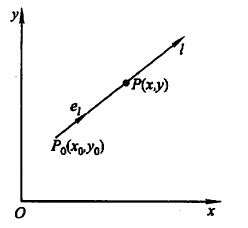射线$l$的参数方程为
$\begin{cases}x=x_0+tcos\alpha ，t\geq0\\ y=y_0+tcos\beta，t\geq0 \end{cases}$
如果函数增量$f(x_0+tcos\alpha,y_0+tcos\beta)-f(x_0,y_0)$与$P$到$P_0$的距离$|PP_0|=t$的比值，当点$P$沿着$l$趋于$P_0(即t \to 0^+)$时极限存在，则称此极限为函数在点$P_0$沿方向$l$的方向导数
$\frac{\partial f}{\partial l}|_{(x_0,y_0)}=\lim \limits_{t \to 0^+}\frac{f(x_0+tcos\alpha,y_0+tcos\beta)-f(x_0,y_0)}{t}$
方向导数与全微分的关系
由全微分的定义得到
$f(x_0+Δx,y_0+Δy)-f(x_0,y_0)=f_x(x_0,y_0)Δx+f_y(x_0,y_0)Δy+o(\sqrt{(Δx)^2+(Δy)^2})$
设点$(x_0+Δx,y_0+Δy)$在以$(x_0,y_0)$为起点的射线$l(cos\alpha,cos\beta是l的方向余弦)$上，则有$Δx=tcos\alpha$,$Δy=tcos\beta$,$\sqrt{(Δx)^2+(Δy)^2}=t$，所以
$\lim \limits_{t \to 0^+}\frac{f(x_0+Δx,y_0+Δy)-f(x_0,y_0)}{t}=f_x(x_0,y_0)cos\alpha+f_y(x_0,y_0)cos\beta$
上式左侧就是方向导数定义形式，极限存在即方向导数存在，且其值等于右式
由此得到定理，如果函数$f(x,y)$在点$P_0(x_0,y_0)$可微分，那么函数在该点沿任一方向$l$的方向导数存在
$\frac{\partial f}{\partial l}|_{(x_0,y_0)}=f_x(x_0,y_0)cos\alpha+f_y(x_0,y_0)cos\beta$
梯度
在平面上确定某一点可能存在无数个方向导数，我们怎样找到其中一个方向导数来描述函数最大变化率？
定义：在二元函数的情形，	设函数$f(x,y)$在平面区域D内具有一阶连续偏导数，对于每一点$P_0(x_0,y_0)\in D$，都可以给出一个向量
$f_x(x_0,y_0)i+f_y(x_0,y_0)j \quad 或用坐标表示 \quad (f_x(x_0,y_0),f_y(x_0,y_0))$
其中$i,j$为$x,y$轴的方向向量，上述微量称为函数$f(x,y)$在点$P_0(x_0,y_0)$的梯度记作
$gradf(x_0,y_0)=f_x(x_0,y_0)i+f_y(x_0,y_0)j$
由定义看到，梯度的方向是确定的，如果点$P$的坐标确定，那么梯度也大小也确定
如果函数$f(x,y)$在点$P_0(x_0,y_0)$可微分，$e_l=(cos\alpha,cos\beta)$是方向$l$的方向向量(方向未确定)
$\frac{\partial f}{\partial l}|_{(x_0,y_0)}=f_x(x_0,y_0)cos\alpha+f_y(x_0,y_0)cos\beta=grad\ f(x_0,y_0).e_l=|grad\ f(x_0,y_0)|cos\theta$
其中$\theta$为向量${grad\ f(x_0,y_0)}$与向量$e_l$的夹角，当$\theta=0$时，即方向$e_l$与梯度${grad\ f(x_0,y_0)}$的方向时，函数$f(x,y)$增加最快，函数在这个方向的方向导数达到最大值，这个值就是梯度${grad\ f(x_0,y_0)}$的模，即
$\frac{\partial f}{\partial l}|_{(x_0,y_0)}=|grad \ f(x_0,y_0)|$
所以可以用沿梯度方向的方向导数来描述是函数最大变化率，即梯度方向是函数变化率最大的方向，在梯度定义的时候就已经赋予了它这个特性。


展开全文高等数学 机器学习 梯度
• 1.导数 代数意义  导数是对一个变量求导,另一个变量当做数 对x求导的话y就看作一个数,描述的是x方向上的变化率 对y求导的话x就看作一个数,描述的是y方向上的变化率 几何意义 对x求导是曲面z=f(x,y)在x...
1.偏导数

代数意义
偏导数是对一个变量求导,另一个变量当做数
对x求偏导的话y就看作一个数,描述的是x方向上的变化率
对y求偏导的话x就看作一个数,描述的是y方向上的变化率

几何意义
对x求偏导是曲面z=f(x,y)在x方向上的切线
对y求偏导是曲面z=f(x,y)在x方向上的切线
这里在补充点.就是因为偏导数只能描述x方向或y方向上的变化情况,但是我们要了解各个方向上的情况,所以后面有方向导数的概念.

2.微分

偏增量：x增加时f(x,y)增量或y增加时f(x,y)
偏微分：在detax趋进于0时偏增量的线性主要部分
detaz=fx（x,y）detax+o(detax)
右边等式第一项就是线性主要部分,就叫做在（x,y）点对x的偏微分
这个等式也给出了求偏微分的方法,就是用求x的偏导数求偏微分
全增量：x,y都增加时f(x,y)的增量
全微分：根号(detax方+detay方）趋于0时,全增量的线性主要部分
同样也有求全微分公式,也建立了全微分和偏导数的关系
dz=Adx+Bdy 其中A就是对x求偏导,B就是对y求偏导
希望楼主注意的是导数和微分是两个概念,他们之间的关系就是上面所说的公式.概念上先有导数,再有微分,然后有了导数和微分的关系公式,公式同时也指明了求微分的方法.

3.全导数

全导数是在复合函数中的概念,和上面的概念不是一个系统,要分开.
u=a(t),v=b(t)
z=f[a(t),b(t)]
dz/dt 就是全导数,这是复合函数求导中的一种情况,只有这时才有全导数的概念.
dz/dt=(偏z/偏u)(du/dt)+(偏z/偏v)(dv/dt)
建议楼主在复合函数求导这里好好看看书,这里分为3种情况.1.中间变量一元就是上面的情况,才有全导数的概念.2.中间变量有多元,只能求偏导 3.中间变两有一元也有多元,还是求偏导.
对于你的题能求对x的偏导数,对y的偏导数,z的全微分,不能求全导数
如果z=f(x^2,2^x) 只有这种情况下dz/dx才是全导数!

展开全文• 3.微分与导数的关系 4.导 类比于一元函数，也想研究函数的变化率问题，在日常生活中，我们经常遇到这样的问题，一个值和许多元素相关，我们习惯只改变一个变量值，其它变量值固定，看变化的情况。 这
• 【微积分5多元函数微分学】第五章第二节 导数与全微分的计算第二节 导数与全微分的计算1. 内容要点1. 复合函数求导2. 隐函数求导2. 常考题型1. 求一点处的导数与全微分2. 求已经给出具体表达式的导数与全...数据结构 leetcode python 人工智能 lambda
• 我们已经知道，二元函数对某个自变量的导数表示当其中一个自...一元函数的情形类似，我们也希望利用关于自变量增量的线性函数来近似地代替函数的全增量，由此引入关于二元函数全微分的定义．由此看出，导数...
• 而f（x、y、z…）的全微分等于f（x、y、z…）在x、y、z…各方向上的导数之和，也就是f（x、y、z…）在各方向的最大增长量之和； 于是当梯度等于向量 N（f对x的导，f对y的导，f对z的导……）时，梯度所对应的...数学
• 复习内容 科目 内容 补充 时间 ...第二节 导数与全微分的计算 ...题型一 求一点出的导数 ...题型二 求已给出具体表达式函数的与全微分 题型三 含有抽象函数的复合函数的与全微分 ...
• 导数与微分 讨论的函数只有一个自变量，这种函数叫做一元函数，但很多实际问题往往牵涉到多方面的因素，反映到数学上，就是一个变量依赖于多个变量，这就提出了多元函数的微分问题。比如圆柱体的体积 和它的底半径 ,...
• 首先关于边界有两种情况，一是包含，二是不包含，包含的叫闭区域，不包含的叫开区域（然后关于区域这个名词，这里说是开区域闭区域的统称，但在复变函数中区域单指开区域，所以，呃），然后看一下内点的概念，所谓...
• 一、偏导数的定义、基本计算方法及几何意义1、偏增量与偏导数的定义2、多元显函数偏导数的计算3、偏导数的几何意义二、高阶偏导数的...与偏微分四、全微分的几何意义及应用1、曲面的切平面与全微分的几何意义2、全微...
• 全微分 　《数学笔记11——微分和不定积分》中说明了什么是一元函数的微分，类似地，在多元函数中同样存在微分的概念，它有一个确切的名字——全微分。 　《多变量微积分笔记1——导数》中，曾经提到过近似，...
• 1.全微分的定义设函数在点的某邻域内有定义.若函数在点处的全增量可表示为其中是仅点有关,而无关的常数,.则称函数在点处可微分;并称线性函数为函数在点处的全微分,记作 ,即对于二元函数,规定自变量的增量为自...
• §8.1 多元函数的基本...建议同学们在学习中，注意将二元函数的概念结论一元函数的相应的概念结论加以比较，区别并理解二者之间的“同中之异，异中之同”，这样会大大地提高学习效率。 一、区域 1、邻域 设是高等数学
• 这几天突然想到了优化理论中的梯度下降算法，看到了几个名词，愣了一下，虽然...数学中的全微分(方程)，全导数(公式)，偏微分(方程)，梯度，导数，方向导数，切线，斜率，射线，可导连续，(多元)函数的积分微分,...
• 基础数学4：导数、导数、方向导数、梯度、全微分回顾 （1）导数： ①导数的定义： 导数代表自变量的变换趋于无穷小时，函数值变化自变量变化的比值，反映函数在某一点处沿x方向的变化率。 在一元函数中只...梯度
• 大家好，我今天讲解的题目是 “二元函数的全微分求积”前面我们学习了两类曲线积分，以及曲线积分和重积分之间的桥梁，我们称之为格林公式，这个公式给出了平面闭区域上的重积分和分段光滑的正向边界曲线的坐标积分...
• 上述二式的左端分别称之为二元函数对或的偏增量，而右端称之为二元函数对或的偏微分。 为了研究多元函数中各个自变量都取得增量时，因变量所获得的增量，即增量的问题，我们先给出函数的增量的概念。 【定义...高等数学
• 1.平面空间直线 对于平面来说有两种方程： 已知点(x0,y0,z0)(x_0,y_0,z_0)(x0​,y0​,z0​) 平面法向量（A,B,C） 平面的点法式方程： A(x−x0)+B(y−y0)+C(z−z0)=0A(x-x_0)+B(y-y_0)+C(z-z_0) = 0A(x−x0​)+B...高等数学
• 龙源期刊网http://www.qikan.com.cn二元函数连续、导数和全微分之间的关系作者：张...全微分DOI：10.16640/j.cnki.37-1222/t.2019.12.202對于一元函数来讲，连续、导数和微分之间的关系比较简单：可导可微是等价...
• 复合函数的导数（锁链法则或链法则） 在 处的导数公式为： （其他复合类型的导数...一阶全微分形式的不变性若有复合函数 ，则其在点 的全微分为： （显然当 为自变量，函数 的全微分同样为 ）全微分公式( 是 ...
• 全微分：https://www.zhihu.com/question/31464934/answer/152231899导数、全导数：什么是全导数？方向导数、梯度：https://www.zhihu.com/question/36301367/answer/156102040（自己看的）(x,y)的导数：过点(x,y)...
• 3. 二元函数的微分（偏增量，偏微分增量） 4. 二元函数的切向量、切平面（假设二元函数具有一阶连续偏导数） 5. 二元函数的局部线性近似（局部线性化函数） 6. 局部...
• 鉴于现有方法不能很好恢复场景深度变化较大的图像,本文融合大气衰减模型变分偏微分方程,提出了一种单幅彩色图像去雾算法。该算法利用数学形态学中的中值集算子构造局部白平衡处理,精确估计大气光参数。设计了一种...研究论文
• 前一篇内容使用了大篇幅介绍一些基本概念，本篇则正式进入多元函数微分学的内容。 回顾 本篇内容为导数，在学习多元函数导数之前，先回顾一下一元函数的导数 二元函数导数定义 ...增量与全增量 ...
• 01考试内容多元函数的概念、二元函数的几何意义、二元函数的极限连续的概念、有界闭区域上多元连续函数的性质、多元函数的导数和全微分全微分存在的必要条件和充分条件 、多元复合函数、隐函数的求导法、二阶...
• 主要内容部分讲课内容截图，视频内容更全面9.2_1 导数的定义及求导方法9.2_2 导数的几何意义高阶导数9.3_1 全微分定义9.3_2 可微的必要条件充分条件9.3_3 全微分的计算9.4_1 多元复合函数求导9.4_2 多元......